## How to find the top 5 or n most frequent text or numbers in Excel?

It is a common task to get the most frequent text from a list of cells for most of us. In certain case, you may also need to find and extract the top 3 or n most frequent text from a list as below screenshot shown. To solve this problem, this article will introduce some quick tricks for you.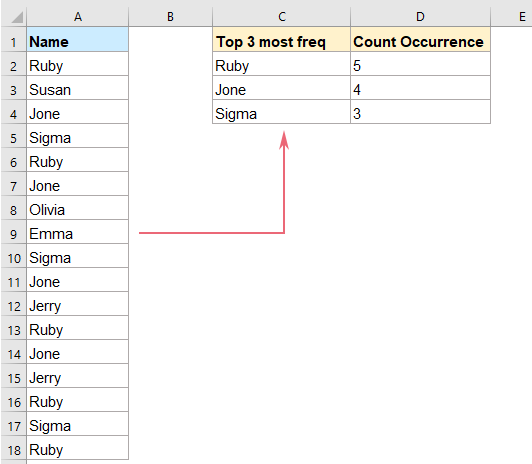#### Find and extract top 3 or n most frequent text or numbers with formulas

You can achieve this task by using the below formulas, please do as this:

##### Formula 1: Normal formula + Array formula

1. Please apply the below formula to get the value that appears the most frequently:

=IFERROR(INDEX(\$A\$2:\$A\$18,MODE(MATCH(\$A\$2:\$A\$18,\$A\$2:\$A\$18,0)+{0,0})),"")

Notes:

1. In this formula, A2:A18 is the data list that you want to find the values from.

2. If you are using the Excel versions that are prior to Microsoft 365, you should press Ctrl + Shift + Enter to get the result. For the users of Microsoft 365 and newer versions of Excel, you can just enter the formula in the result cell, and then press Enter key.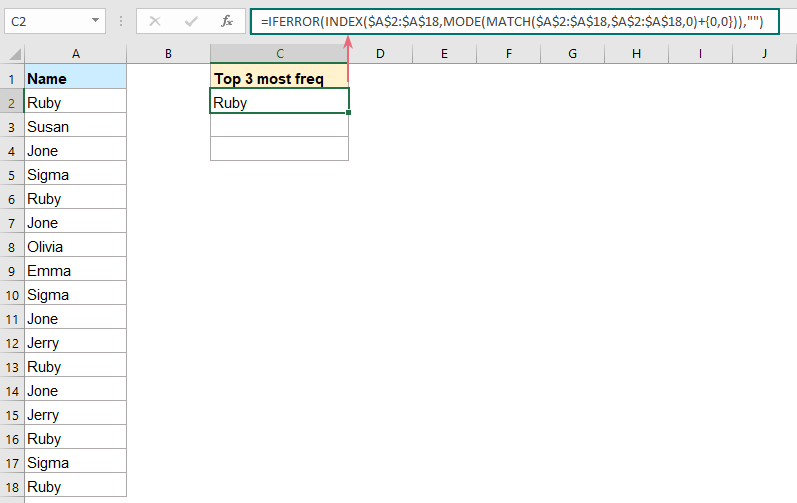2. Then, you should use the following array formula to get the second most frequent value, enter this formula below the first formula cell, and then press Ctrl + Shift + Enter keys together to get the correct result, see screenshot:

=IFERROR(INDEX(A\$2:A\$18,MODE(IF(COUNTIF(C\$2:C2,A\$2:A\$18)=0,MATCH(A\$2:A\$18,A\$2:A\$18,0)+{0,0}))),"")
Note: In this formula, A2:A18 is the data list that you want to find the values from, C2 is the formula cell of the most frequent value you have extracted just.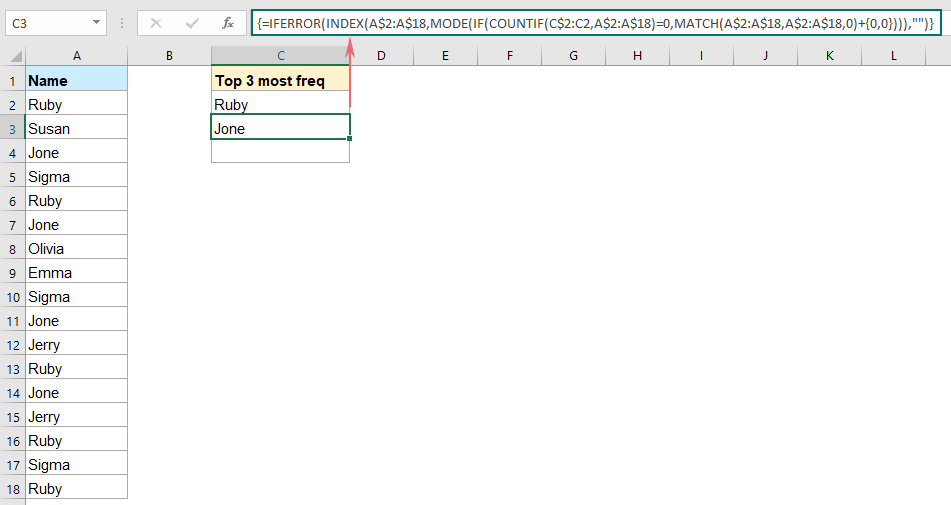3. After extracting the second most frequent value, drag this formula cell down to get other n values as you need. See screenshot: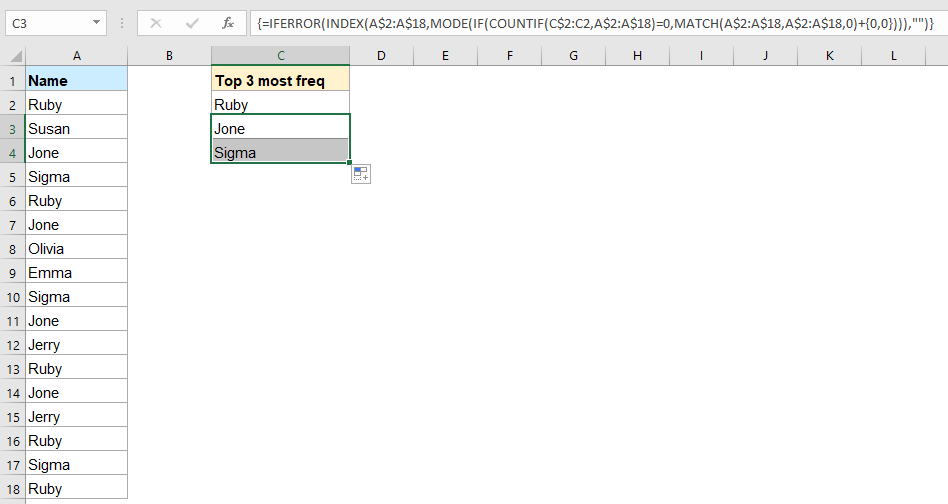4. Finally, you can use the following formula to get the number of occurrences of the extracting text, see screenshot:

=COUNTIF(\$A\$2:\$A\$18, C2)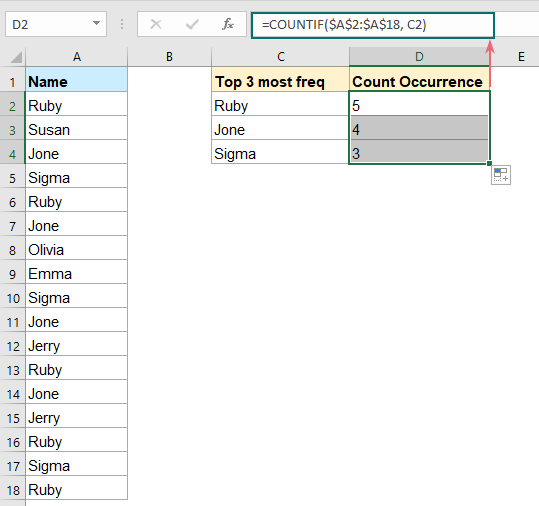##### Formula 2: Array formula

In fact, you can also use a single array formula to get the result, please do as this:

1. Enter or copy the below formula into a blank cell where you want to get the result, then, press Ctrl + Shift + Enter keys together to get the first result, see screenshot:

=INDEX(\$A\$2:\$A\$18,MATCH(MIN(MODE(IF(NOT(COUNTIF(C\$1:C1,\$A\$2:\$A\$18)),(COUNTIF(\$A\$2:\$A\$18,"<"& \$A\$2:\$A\$18)+1)*{1,1}))),COUNTIF(\$A\$2:\$A\$18,"<"& \$A\$2:\$A\$18)+{1},0))
Note: In the formula, A2:A18 is the data list that you want to find the values from, C1 is the above cell of your formula.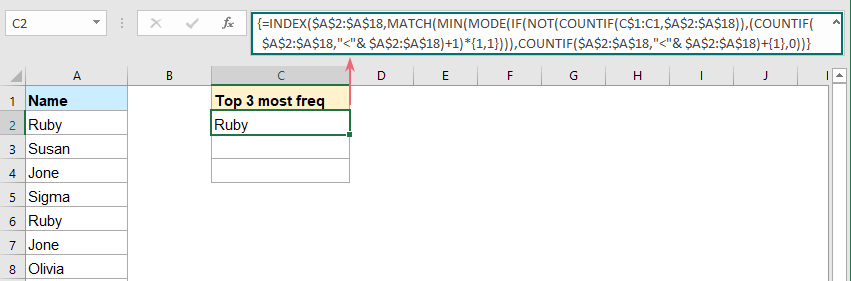2. And then, drag this formula down to get other values. If you want to get the top 3 most frequent values, you just need to drag down to other two cells, see screenshot: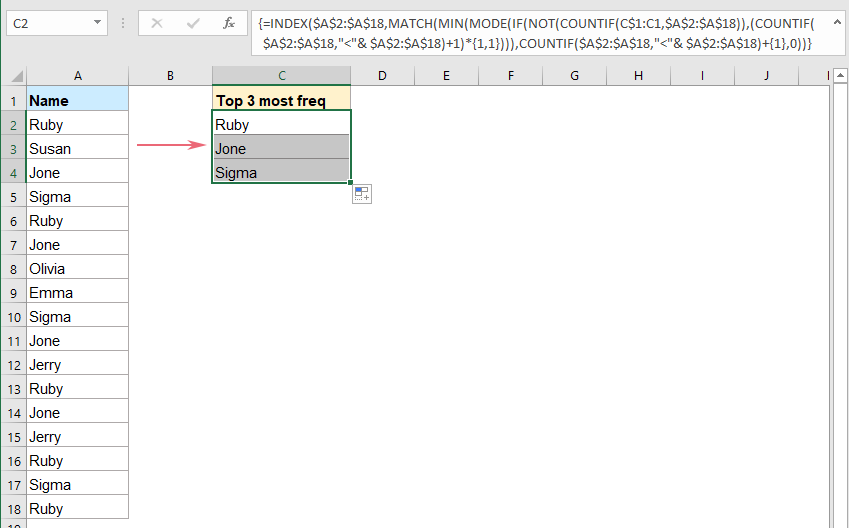3. As well, you can also get the number of occurrences of the extracting text with below formula:

=COUNTIF(\$A\$2:\$A\$18, C2)#### Find and extract top 3 or n most frequent text or numbers with Pivot Table

If you are familiar with PivotTable, you can quickly get a table which arranges the data from the most frequently occurring to least frequently occurring. Please do with the following steps:

1. Select the data list that you want to use, then, click Insert > PivotTable, see screenshot: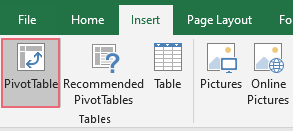2. In the popped out Create PivotTable dialog box, select the place where you want to put the PivotTable from the Choose where you want the PivotTable report to be placed section, see screenshot: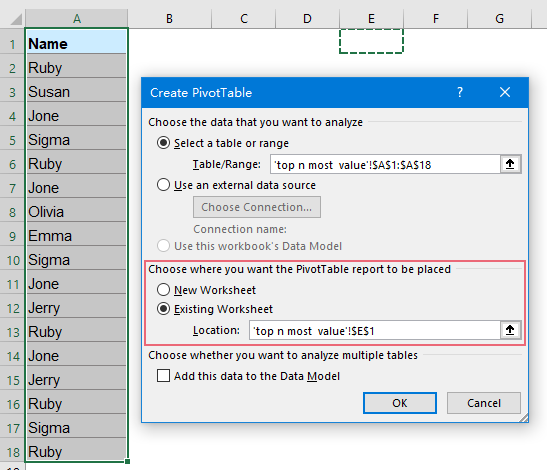3. Then, click OK button, a PivotTable Fields pane is displayed. Drag the column name into the Rows and Values areas separately, and you will get a pivottable as below screenshot shown: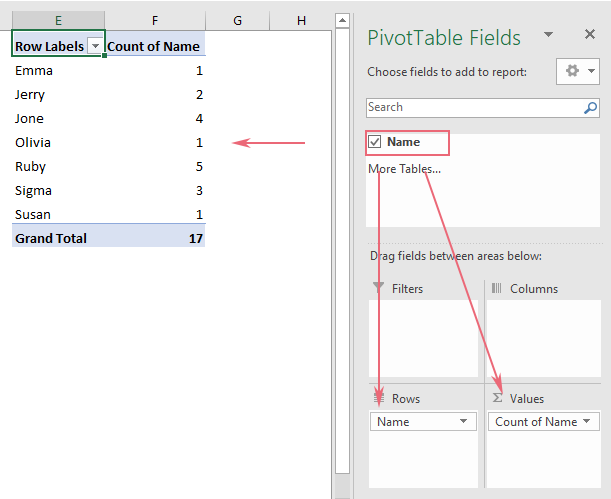4. Click any cell in the number column, then, right click, and choose Sort > Sort Largest to Smallest, see screenshot: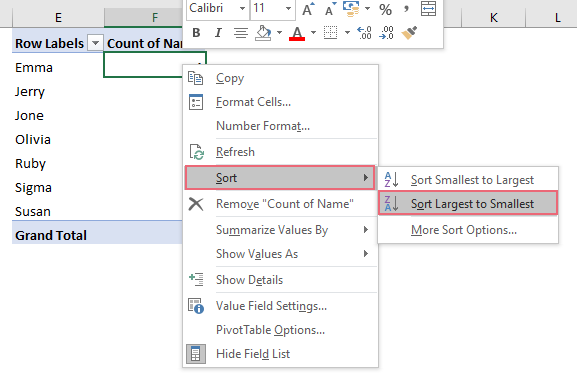5. And then, the count of values in the pivotable are sorted from largest to smallest, and you can see the top 3 most frequent texts at glance, see screenshot: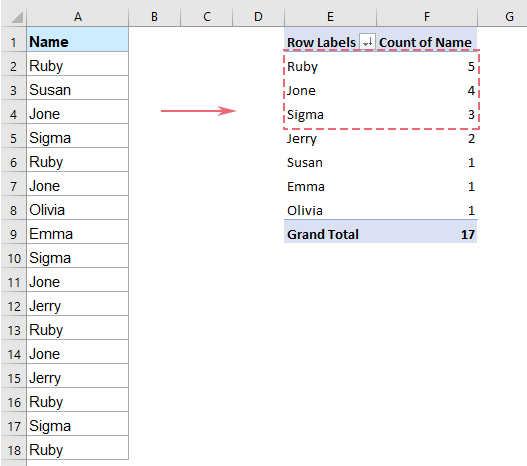#### More relative articles:

• Find Common Values In 3 Columns
• In general case, you may need to find and select the same values between two columns in Excel, but, have you ever tried to find the common values among three columns which means the values exist in 3 columns at the same time as following screenshot shown. This article, I will introduce some methods to finish this task in Excel.
• Find The Most Frequent Text With Criteria
• This article will talk about how to find the most occurred text based on the specific criteria from a range of cells in Excel. At the same time, I will also introduce the formula for extracting the most frequently occurring text in a column.
• Sort Data By The Most Frequent Value
• Supposing you have a long list of data in your worksheet, and now you would like to sort this list by the frequency of which each word occurs. That is to say, the most common value (for example, occurs four times in the column) is listed first, and then be followed by the words which occur three times, twice and once as following screenshots shown. How could you solve this task in Excel?
• Find The Least Or Most Common / Frequent Value
• When you work on Excel workbook, sometimes, you may need to find the least common or frequent value in a column or a row of the worksheet. Excepting find it one by one manually, there is a formula which combine the Index, Match, Min and Countif functions can quickly return the least frequently occurring value.

### Best Office Productivity Tools

 Popular Features: Find, Highlight or Identify Duplicates   |  Delete Blank Rows   |  Combine Columns or Cells without Losing Data   |   Round without Formula ... Super Lookup: Multiple Criteria VLookup  |   Multiple Value VLookup  |   VLookup Across Multiple Sheets   |   Fuzzy Lookup .... Advanced Drop-down List: Quickly Create Drop Down List   |  Dependent Drop Down List   |  Multi-select Drop Down List .... Column Manager: Add a Specific Number of Columns  |  Move Columns  |  Toggle Visibility Status of Hidden Columns  |  Compare Ranges & Columns ... Featured Features: Grid Focus   |  Design View   |   Big Formula Bar   |  Workbook & Sheet Manager   |  Resource Library (Auto Text)   |  Date Picker   |  Combine Worksheets   |  Encrypt/Decrypt Cells   |  Send Emails by List   |  Super Filter   |   Special Filter (filter bold/italic/strikethrough...) ... Top 15 Toolsets:  12 Text Tools (Add Text, Remove Characters, ...)   |   50+ Chart Types (Gantt Chart, ...)   |   40+ Practical Formulas (Calculate age based on birthday, ...)   |   19 Insertion Tools (Insert QR Code, Insert Picture from Path, ...)   |   12 Conversion Tools (Numbers to Words, Currency Conversion, ...)   |   7 Merge & Split Tools (Advanced Combine Rows, Split Cells, ...)   |   ... and more

Supercharge Your Excel Skills with Kutools for Excel, and Experience Efficiency Like Never Before. Kutools for Excel Offers Over 300 Advanced Features to Boost Productivity and Save Time.  Click Here to Get The Feature You Need The Most...#### Office Tab Brings Tabbed interface to Office, and Make Your Work Much Easier

• Enable tabbed editing and reading in Word, Excel, PowerPoint, Publisher, Access, Visio and Project.
• Open and create multiple documents in new tabs of the same window, rather than in new windows.
• Increases your productivity by 50%, and reduces hundreds of mouse clicks for you every day!